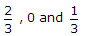# Civil Engineering - UPSC Civil Service Exam Questions

11.

Consider the following factors :
1. Period of construction, winter/summer
2. Degree of foundation roughness
3. Slab thickness
4. Reinforced/unreinforced
Which of these factors are considered as per IRC for obtaining the maximum expansion joint spacing in rigid pavements ?

 A. 1, 2 and 3 B. 2, 3 and 4 C. 2 and 3 D. 1 and 4

Explanation:

No answer description available for this question. Let us discuss.

12.

The concentration of hardness producing cations may be estimated using which one of the following ?

 A. Conductivity meter B. pH meter C. Spectrophotometer D. Flame photometer

Explanation:

No answer description available for this question. Let us discuss.

13.

A vehicle is moving up on an inclined plane. The vehicle has rear-wheel drive. Force of friction on the rear wheels will be in a direction

 A. along the plane but backward B. backward at an angle φ with the plane, where φ is the friction angle C. along the plane but forward D. forward at an angle φ with the plane, where φ is the friction angle

Explanation:

No answer description available for this question. Let us discuss.

14.

Consider the following statements :
In the case of close coiled spring carrying an axial loading the spring is subjected to :
1. torsion
2. axial force
3. bending moment the effect of which can be neglected.
Of these statements :

 A. 1, 2 and 3 are correct B. 1 and 2 are correct C. 1 and 3 are correct D. 2 and 3 are correct

Explanation:

No answer description available for this question. Let us discuss.

15.

The given figure shows a frame to be analysed by moment distribution method. The distribution factors for members GF, GH and GD will be respectively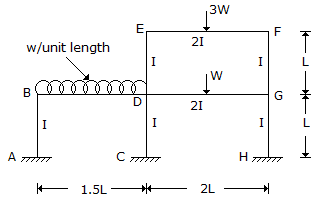A.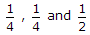B.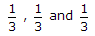C.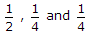D.